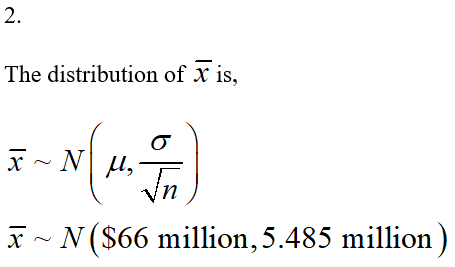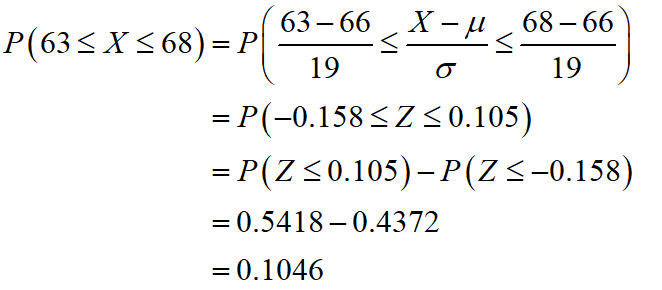# The average production cost for major movies is 66 million dollars and the standard deviation is 19 million dollars. Assume the production cost distribution is normal. Suppose that 12 randomly selected major movies are researched. Answer the following questions.  Give your answers in millions of dollars, not dollars.  Round all answers to 4 decimal places where possible.What is the distribution of XX? XX ~ N(,)What is the distribution of ¯xx¯? ¯xx¯ ~ N(,)For a single randomly selected movie, find the probability that this movie's production cost is between 63 and 68 million dollars. For the group of 12 movies, find the probability that the average production cost is between 63 and 68 million dollars. For part d), is the assumption of normal necessary? YesNo

Question
73 views

The average production cost for major movies is 66 million dollars and the standard deviation is 19 million dollars. Assume the production cost distribution is normal. Suppose that 12 randomly selected major movies are researched. Answer the following questions.  Give your answers in millions of dollars, not dollars.  Round all answers to 4 decimal places where possible.

1. What is the distribution of XX? XX ~ N(,)
2. What is the distribution of ¯xx¯? ¯xx¯ ~ N(,)
3. For a single randomly selected movie, find the probability that this movie's production cost is between 63 and 68 million dollars.
4. For the group of 12 movies, find the probability that the average production cost is between 63 and 68 million dollars.
5. For part d), is the assumption of normal necessary? YesNo
check_circle

Step 1

1.

The distribution of X is, X~N(\$66 million, \$19 million).

Step 2Step 3

3.

The probability that one randomly selected movie's production cost is between 63 and 68 million dollars is,Thus, the probability that one randomly selected movie's production cost is between 63 and 68 million dollars is 0.1046.

...

### Want to see the full answer?

See Solution

#### Want to see this answer and more?

Solutions are written by subject experts who are available 24/7. Questions are typically answered within 1 hour.*

See Solution
*Response times may vary by subject and question.
Tagged in